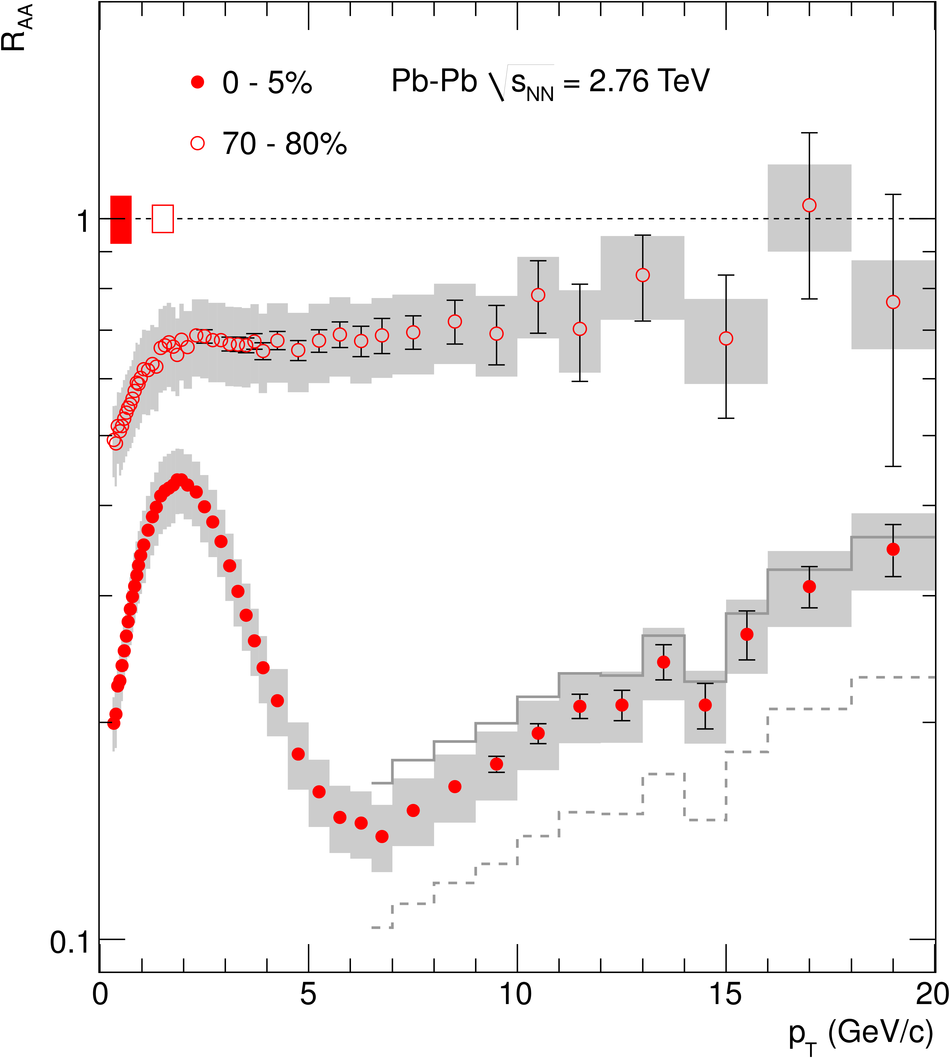# Figure 3

 $R_{AA}$ in central (0--5%) and peripheral (70--80%) Pb--Pb collisions at $\sqrt{s_{_{NN}}} =2.76$ TeV. Error bars indicate the statistical uncertainties. The boxes contain the systematic errors in the data and the $p_T$ dependent systematic errors on the pp reference, added in quadrature. The histograms indicate, for central collisions only, the result for$R_{AA}$ at $p_T>6.5$ GeV/$c$ using alternative pp references obtained by the use of the p$\bar{\rm p}$measurement at $\sqrt{s_{_{NN}}}=1.96$ TeV  in the interpolation procedure (solid) and by applying NLO scaling to the pp data at 0.9 TeV (dashed) (see text) The vertical bars around $R_{AA}=1$ show the $p_T$ independent uncertainty on $\langle N_{\rm{ coll}} \rangle$